## Saturday, July 30, 2016

### Convexity Checker

In this post a somewhat complex class of functions was incorrectly assumed to be convex: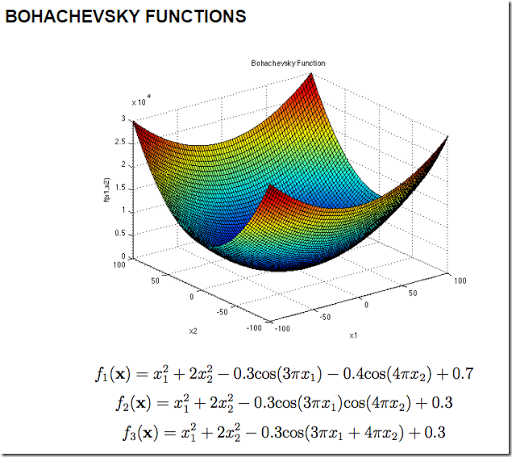(From: http://www.sfu.ca/~ssurjano/boha.html, the plot is $$f_1(x)$$).

Indeed the picture gives the impression this is a simple bowl shaped thing and should be easy to minimize. Most functions with a cosine term are not convex, and indeed we can see more details here:(From: http://al-roomi.org/benchmarks/unconstrained/2-dimensions/11-bohachevsky-s-function-no-2; this plot is $$f_2(x)$$).

All the functions have a global optimal solution $$x^*_1=0$$, $$x^*_2=0$$, $$f^*=0$$.

#### Solving with multi-start local solver

It is not so difficult to solve this with a good local solver: use a simple multi-start strategy. I.e. using the NLP solver CONOPT and 20 trials with a random starting point $$x^0_1,x^0_2 \in [-100,100]$$ gives:

 ----     27 PARAMETER xsol                  x.1         x.2       f.obj trial1       0.6186 1.35041E-22      0.4129trial2       0.6186 -5.9644E-14      0.4129trial3  -8.6989E-15 -2.9675E-11trial4  5.69333E-16     -0.4695      0.4699trial5       0.6186 7.34013E-24      0.4129trial6      -0.6186      0.4695      0.8828trial7  -3.6954E-14 -2.5271E-18trial8      -1.2225      0.9334      3.5184trial9      -0.6186 2.67223E-16      0.4129trial10     -0.6186 -6.5540E-18      0.4129trial11     -0.6186 -3.0311E-23      0.4129trial12 -3.5326E-14 -6.9878E-11trial13 -1.6458E-10 -1.1308E-11trial14 -5.1330E-11     -0.4695      0.4699trial15      0.6186     -0.4695      0.8828trial16      0.6186      0.4695      0.8828trial17 -1.9055E-12 2.87931E-12trial18     -0.6186 -1.4977E-14      0.4129trial19      0.6186 -7.2286E-10      0.4129trial20 -2.5755E-16 8.27181E-24

(blank means zero in this GAMS output). There was no problem in finding the global optimum $$x^*_1=0$$ , $$x^*_2=0$$ , $$f^*=0$$ here.

#### Solving with a global solver

Not all solvers support the $$cos(x)$$ function:

1. Baron: Cannot handle function 'cos'
2. Couenne: found global optimum
3. Antigone: ERROR: Unsupported function cos in objective defObj_f

#### Tools to verify convexity

It would be nice if we had a tool that could detect convexity. A modeling system like AMPL and GAMS has knowledge about the analytical form of the optimization problem, so in theory it could try to find out if the problem is convex. This is not a trivial task (see (1)). A global solver like Baron does in essence a similar thing before solving the problem. A more heuristic approach is used in (2).

#### References

1. R. Fourer, C. Maheshwari, A. Neumaier, D. Orban and H. Schichl, “Convexity and Concavity Detection in Computational Graphs: Tree Walks for Convexity Assessment.”  INFORMS Journal on Computing 22(2010) 26–43.
2. John W. Chinneck (2002), “Discovering the Characteristics of Mathematical Programs via Sampling, Optimization Methods and Software, vol. 17, no.2, pp. 319-352

## Monday, July 25, 2016

### Excel Pivot Table and large data sets: PowerPivotIn this post I described a simple way to export a large GAMS data set to a CSV file which can be used as an external data source by Pivot Tables. Basically this was needed as Excel has a limit of a million rows, so we cannot store the data in Excel itself as a table. However newer versions of Excel have a facility called PowerPivot that allows us to store a very large data (or even multiple tables) in what is called a “data model”. This is an internal memory based database (“in-memory analytics engine”), and it does not have a strict row limit. Subsequently, we can create a Pivot Table based on this data. Here is how to set this up interactively.

### Recipe

1. Write the GAMS data to a GDX file. The easiest is to create a single large multi-dimensional “Cube” parameter.
2. Call GDXDUMP to create a CSV file.
3. In Excel 2016 use New Query in the Data tab to import the CSV file.4. In the Query Editor select “Close & Load To".5. In the dialog box unselect “Table” and select “Add this data to the Data Model”.6. After pressing the Load button you will see something like:We are just exceeding the Excel row limit, but no sweat here.
7. In the Insert tab, select PivotTable. Use the Data Model as data source.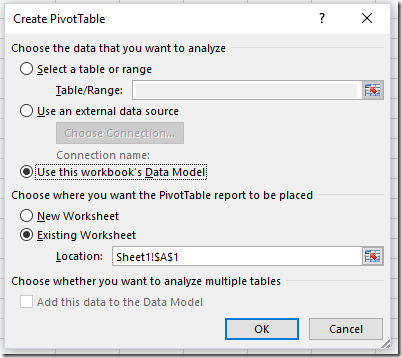8. After OK we have our pivot table: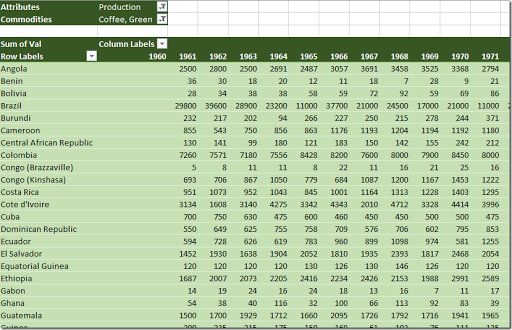#### Limits

The limits are discussed here.

The .xlsx file containing the data can become large. After reading a the above cube.csv file (63 MB) the resulting .xlsx file is only 3 MB. So the compression may actually be doing very well.

The row limit of a table in the data model seems to be: 1,999,999,997, well beyond the row limit of Excel sheets.

#### Notes:

1. PowerPivot/PowerQuery is fairly new. I encountered some crashes of Excel when using more advanced features.
3. Not sure how the compatibility works out. Can I send this spreadsheet to anyone with Excel 2016? (Or 2013?)
4. I tried quickly to move these steps into a VB script. That did not really work. The generated VBA code by the macro recorder is somewhat ugly for these Power Query calls. May be I should try again but then pay more attention to the details.
5. The pivot table seems more responsive than with the external data source. Having all in-memory helps.
6. Advantage of having the data embedded: .xslx file is self-contained. With external data source one needs to ship several files.
7. With the option Manage Data Model in the Data tab we can look at the tables stored in the data model and perform operations. This is not an Excel table and the syntax for formulas is different (using a language called DAX – Data Analysis Expressions). See here.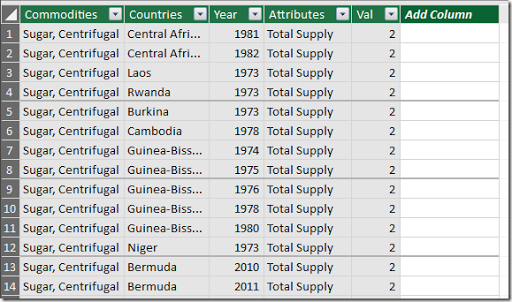## Sunday, July 24, 2016

### Non-convex QP as a MIP: performance

In this post I showed how a small non-convex QP could be reformulated as a MIP using KKT conditions plus a special (linear) objective. Will this work in practice? How does this compare performance-wise with global solvers? Let’s see if we can get a feeling for this.

#### Duplicating the problem

One easy way to make the small test problem bigger is to duplicate the problem:

 Objective $$c'x_1 + x'_1 Q x_1$$ $$+c'x_2 + x'_2 Q x_2$$ $$\cdots$$ $$+c'x_K + x'_K Q x_K$$ Constraints $$A x_1 \le b$$$$\ell \le x_1 \le u$$ $$A x_2 \le b$$$$\ell \le x_2 \le u$$ $$\ddots$$ $$A x_K \le b$$$$\ell \le x_K \le u$$
This way we can make the model easily much bigger by introducing a set K. Notice that solvers have a difficult time to see through this trick. They are not recognizing they are solving a tiny problem that is just repeated K times. Actually these independent blocks often make the problem tough to solve (I don't know exactly why, but experience shows this is the case).
##### Modeling

Basically we need to add an index K to the variables $$x_i$$, and to the constraints. Finally we add a summation to the objective. The new model looks like: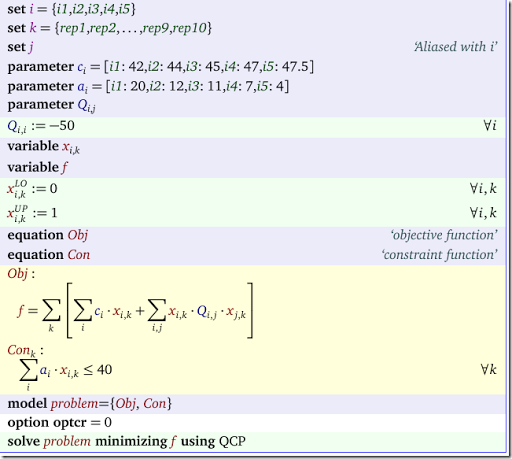For the KKT model we can do something similar.

#### Results

Here are some results with K=5 and K=10. I stopped after 1000 seconds (if not proven optimal by then, I report the gap).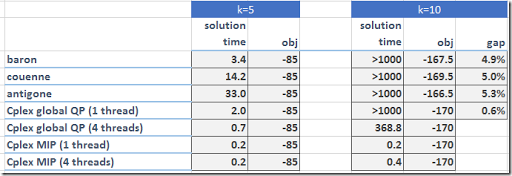Note: The global QP solver GloMiQo behaves the same as Antigone.

At least for this model the MIP formulation is doing very well. The Cplex MIP solver beats the Cplex global QP solver by a large margin!

#### Yalmip

Yalmip has reported similar results produced by its KKTQP method.

## Monday, July 18, 2016

### Non-convex QPs and Marginals

The Cplex global QP solver has some problems providing marginals (duals and reduced costs) for a non-convex QP. Here is a small model taken from (1):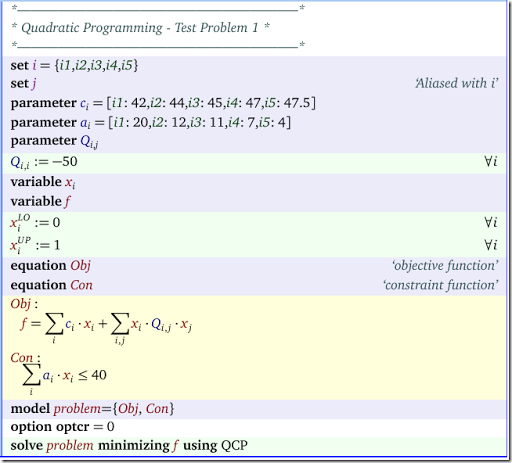To solve this problem to optimality in GAMS we add ‘option optcr=0’ and we provide a Cplex option ‘OptimalityTarget = 3’.

#### Solution

The solution looks like:

 LOWER          LEVEL          UPPER        ---- EQU Obj                 .              .              .                  ---- EQU Con               -INF           39.0000        40.0000                      Obj  objective function  Con  constraint function ---- VAR x            LOWER          LEVEL          UPPER         i1          .             1.0000         1.0000            i2          .             1.0000         1.0000            i3          .              .             1.0000             i4          .             1.0000         1.0000             i5          .              .             1.0000                                        LOWER          LEVEL          UPPER         ---- VAR f                 -INF          -17.0000        +INF

The marginals are missing. Indeed the log contains message:

 *** No duals possible for models with quadratic objective when solved globally.

#### Workarounds

The simplest way to get the marginals of this problem is to use a global solver that provides duals:

These solvers do not provide (correct) duals:

• Cplex
• Couenne
• LindoGlobal (strangely LindoGlobal provides some duals correctly, but others are incorrect).

One way to get marginals with a global solver that does not provide them is resolving the problem with a local solver. As in: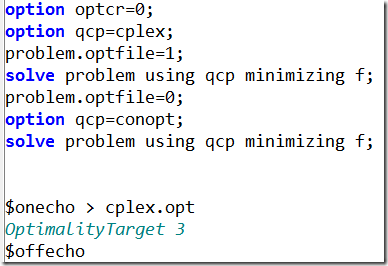For this model it takes Cplex 28 iterations to find the global solution, and then Conopt needs 4 iterations to recover the duals. The results look like:

 LOWER          LEVEL          UPPER         MARGINAL ---- EQU Obj                 .              .              .             1.0000      ---- EQU Con               -INF           39.0000        40.0000          .            Obj  objective function  Con  constraint function ---- VAR x            LOWER          LEVEL          UPPER         MARGINAL i1          .             1.0000         1.0000       -58.0000      i2          .             1.0000         1.0000       -56.0000      i3          .              .             1.0000        45.0000      i4          .             1.0000         1.0000       -53.0000      i5          .              .             1.0000        47.5000                                 LOWER          LEVEL          UPPER         MARGINAL ---- VAR f                 -INF          -17.0000        +INF             .

An alternative is to solve the non-convex QP as a MIP using a model with KKT conditions and a special objective (see here).

#### References

(1)  Floudas e.a., Handbook of Test Problems in Local and Global Optimization, Kluwer, 1999.